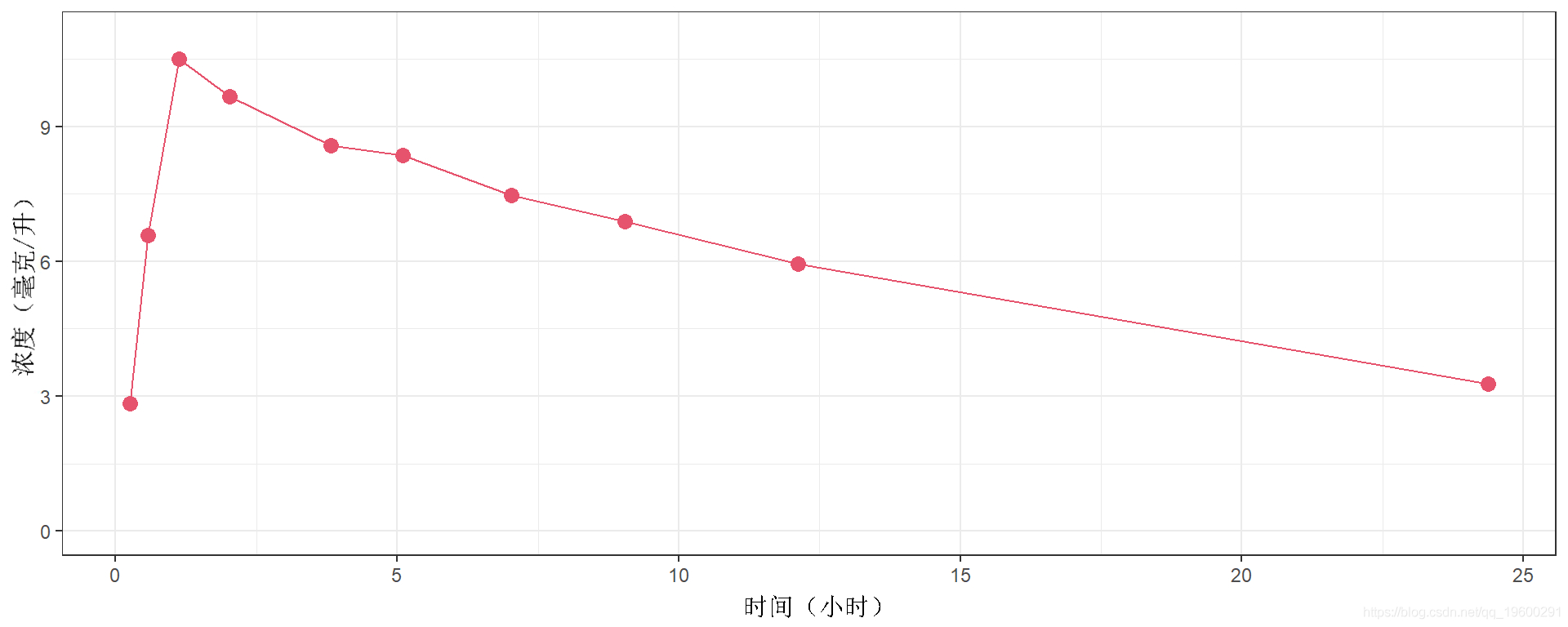# 简介

`head(thdat)`

12 名受试者在时间 0 时接受了 320 mg 茶碱。

`plot(data=theo.data2) +eo_ine(oaes(group=id))`

## 数据的个体差异

`pl + geom\_line() + facet\_wrap(~id)`

# 将非线性模型拟合到数据

## 将非线性模型拟合到单个受试者

``` the.dat.dta\$id==1 ,c("tme)\]
plot(data=teo1```我们可能想为这个数据拟合一个 PK 模型

```pk.od <- function(pi, t){
D  <- 320
ka
V
ke
f  <- D\*a/V/(a-k)\*(exp(-e\*t)-exp(-k\*t))```

# R语言LME4混合效应模型研究教师的受欢迎程度

```nls(neatin ~p.me1(psi, time))
coef(km1)```

```e. <- dafme(tm=sq(0,40,=.2))
w.pd1 <- pedct(pk, newaa=wdf)
line(da=new., aes(x=tie,y=re1))```

## 将独特的非线性模型拟合到几个患者上

` nls(ocetn ~ kme1(ps, tme)`
```nll <- predct(kmll, ewta=n.f)
p+geom_line(ewd,astm,=rdal,clu="390" )```

# 这些估计的 PK 参数是典型的 PK 参数，并且该 PK 曲线是该患者样本的典型 PK 曲线。

`line(data=e.d, aes(x=im,y=pe.al)) + faetap(~ id)`

# 在该模型中，ψi 的最小二乘估计定义为

```for (i in (1:N)) {
pkmi <- nls(cocetatn ~ pk.mdl1(psi, time)
pred <- c(prd, prdit(kmi, neta=ewf))
}```

```nc <- lengh(nwdtie)
tepred <- data.rame(d=rp(1:12),acc),tie=renew.fime12 fpre=pre)
line(dta=te.re, aes(x=me,y=frd)) + factrp(id)```

# 非线性混合效应 (NLME) 模型

i) 使用方程：

ii) 或使用概率分布：

## 对茶碱数据拟合 NLME 模型

```Data(dta       = data,
grp      = id",
prditors = "time",
repose   = "con")```

```molct <- function(pi,id,x) {
D   <- 320
fe <-D\*a/(V\*(a-e))*(exp(-e\*t)-exp(-a\*t))```

```Model(modl = moelpt,
pi  = c(a=1,V=20,ke=0.5))```

```list(map=TRUE,seed=632545)
mix(model, dat,optns)```

`results`

```pred(fit1)
plot.fit(fit1)```

`pltobsv(fit1,lvl=1)`

`pltsateresi(fit1, levl=1)`

## 模型的一些扩展

### 残差模型

`Model(mo=md1p, p0=c(ka=1,V=20,ke=0.5), mdl="constant")`

`Model(modl=dl1pt,error="prori")`

`Model(moel=d1ct, mde="bined")`

`Model( ero.dl="exp")`

### 单个参数的变换

logit 函数定义在 (0,1)上并取其在 RR 中的值：对于 (0,1)中的任何 x，

probit函数是与标准正态分布N(0,1)相关的反累积分布函数（量化函数）ψ-1。对于(0,1)中的任何x。

```Model(model  ,
psi   ,
trns.par = c(1,0,1))```

### 带有协变量的模型

```Model(
trasf   = c(1,0,1),
covri = c(0,1,0))```

Data对象现在需要包括log（wi/70）这个协变量。

```lw70 <- log(weight/70)
Data(data,
res=c("cerato"),
cova=c("lw70"))```

```Model(
trans.pr   = c(1,1,1),
cor = c(0,1,0))```

### 随机效应之间的相关性

```Model(
covai = t(c(0,1,0)),
covain = matrix(c(0,0,0,0,1,1,0,1,1),nrow=3))```

Kaizong Ye拓端研究室（TRL）的研究员。

​非常感谢您阅读本文，如需帮助请联系我们！QQ在线咨询

15121130882

0571-63341498

## 关注有关新文章的微信公众号

This will close in 0 seconds